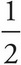# AP Physics 1 Question 220: Answer and Explanation

### Test Information

Question: 220

1. An object of mass 2 kg has a linear momentum of magnitude 6 kg · m/s. What is this object's kinetic energy?

• A. 3 J
• B. 6 J
• C. 9 J
• D. 12 J

The magnitude of the object's linear momentum is p = mv. If p = 6 kg · m/s and m = 2 kg, then v = 3 m/s. Therefore, the object's kinetic energy is K =mv2 =(2 kg)(3 m/s)2 = 9 J.